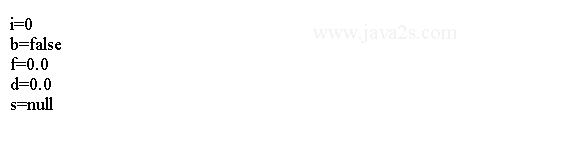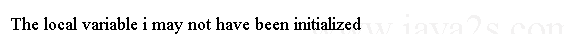# Java Tutorial - Java Class Variables

## Three types of class variables

Java supports variables of three different lifetimes:

• Member variable
• Method local variables
• Static variable

## Class member variable

A member variable of a class is created when an instance is created, and it is destroyed when the object is destroyed. All member variables that are not explicitly assigned a value during declaration are automatically assigned an initial value. The initialization value for member variables depends on the member variable's type.

The following table lists the initialization values for member variables:

Element Type Initial Value
byte 0
short 0
int 0
long 0L
float 0.0f
double 0.0d
char '\u0000'
boolean false
object referencenull

In the following example, the variable x is set to 20 when the variable is declared.

```public class Main{
int x = 20;
}
```

The following example shows the default value if you don't set them.

```class MyClass {//  w w w  .  ja  v a2s. co  m
int i;

boolean b;

float f;

double d;

String s;

public MyClass() {
System.out.println("i=" + i);
System.out.println("b=" + b);
System.out.println("f=" + f);
System.out.println("d=" + d);
System.out.println("s=" + s);
}

}

public class Main {
public static void main(String[] argv) {
new MyClass();

}
}
```

The output:## Example for method local variables

An automatic variable of a method is created on entry to the method and exists only during execution of the method. Automatic variable is accessible only during the execution of that method. (An exception to this rule is inner classes).

Automatic variable(method local variables) are not initialized by the system. Automatic variable must be explicitly initialized before being used. For example, this method will not compile:

```public class Main{
public int wrong() {
int i;
return i+5;
}

}
```

The output when compiling the code above:## Class variable (static variable)

There is only one copy of a class variable, and it exists regardless of the number of instances of the class. Static variables are initialized at class load time; here `y` would be set to 30 when the `Main` class is loaded.

```public class Main{
static int y = 30;
}
```

## Java this Keyword

`this` refers to the current object.

`this` can be used inside any method to refer to the current object.

The following code shows how to use `this` keyword.

```// A use of this.
Rectangle(double w, double h) {
this.width = w; // this is used here
this.height = h;
}
```

## Hidden instance variables and this

Use `this` to reference the hidden instance variables.

Member variables and method parameters may have the same name. Under this situation we can use this to reference the member variables.

```Rectangle(double width, double height) {
this.width = width;
this.height = height;
}
```

The following example shows how to use this to reference instance variable.

```class Person{//  w ww.  java  2  s  . c  o  m
private String name;

public Person(String name) {
this.name = name;
}
public String getName() {
return name;
}
public void setName(String name) {
this.name = name;
}
}
public class Main{
public static void main(String[] args) {
Person person = new Person("Java");
System.out.println(person.getName());
person.setName("new name");
System.out.println(person.getName());
}
}
```

The code above generates the following result.